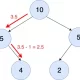2022-11-09
###### How to determine epsilon and MinPts parameters of DBSCAN clustering
2022-12-18

The field of statistics is becoming increasingly important in the world of data science and machine learning. I have recently created a GitHub repository that provides some implementations of statistics topics in Python. You need to have intermediate knowledge of statistics and probability such as population, sample, random variables, etc to be able to follow the content. You can learn about the theories behind the concepts implemented in this repo using my posts on Statistics and Probability.

Here is the link to this repository:

https://github.com/iamirmasoud/statistics_concepts

The structure of repository is organized in such a way that it is easy to navigate and find relevant material. The following are the major concepts that are covered in the repository:

1. Sampling Distributions and Central Limit Theorem
2. Confidence Intervals
3. Hypothesis Testing
4. A/B Testing
5. Linear Regression
6. Multiple Linear Regression
7. Logistic Regression

The Sampling Distributions and Central Limit Theorem section covers the basic concepts of probability and sampling distributions. It explains how to create sampling distributions, the law of large numbers, the central limit theorem, and bootstrapping. These concepts are fundamental to the understanding of statistics and are necessary for more advanced topics.

The Confidence Intervals section covers the concept of confidence intervals and the various methods of constructing them. It explains the difference between traditional confidence intervals and confidence intervals based on bootstrapping.

The Hypothesis Testing section covers the concept of hypothesis testing and the different methods of hypothesis testing. It explains how to use confidence intervals to make decisions, simulate the null hypothesis to make decisions, and simulate the null hypothesis.

The A/B Testing section covers the concept of A/B testing, which is commonly used in marketing to compare the performance of two different web pages or advertising campaigns. The section explains how to design and conduct an A/B test and how to analyze the results.

The Linear Regression section covers the concept of linear regression and the different methods of implementing linear regression. It explains the closed-form solution for linear regression, the interpretation of model coefficients, and the use of linear regression for predicting values.

The Multiple Linear Regression section covers the concept of multiple linear regression, which is an extension of linear regression that involves multiple predictor variables. It explains the interpretation of model coefficients, the use of dummy variables, and the detection of multicollinearity.

The Logistic Regression section covers the concept of logistic regression, which is used for binary classification problems. It explains how to fit a logistic regression model, how to interpret the results, and how to diagnose the model.

The Advanced Topics section covers more advanced concepts such as Bayesian regression, Gibbs sampling, MCMC experiments, and Thompson sampling. These topics are necessary for experts who want to delve deeper into the field of statistics for data science.